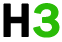C++基础 专题

C++ 预处理器

c++ 还支持很多预处理指令，比如 #include、#define、#if、#else、#line 等，让我们一起看看这些重要指令。

## #define 预处理

#define 预处理指令用于创建符号常量。该符号常量通常称为，指令的一般形式是：

```#define macro-name replacement-text
```

``````#include <iostream>
using namespace std;

#define pi 3.14159

int main ()
{

cout << "value of pi :" << pi << endl;

return 0;
}``````

```\$gcc -e test.cpp > test.p

...
int main ()
{

cout << "value of pi :" << 3.14159 << endl;

return 0;
}```

## 函数宏

``````#include <iostream>
using namespace std;

#define min(a,b) (((a)<(b)) ? a : b)

int main ()
{
int i, j;
i = 100;
j = 30;
cout <<"the minimum is " << min(i, j) << endl;

return 0;
}``````

```the minimum is 30
```

## 条件编译

```#ifndef null
#define null 0
#endif
```

```#ifdef debug
cerr <<"variable x = " << x << endl; #endif ```

```#if 0
不进行编译的代码
#endif
```

``````#include <iostream>
using namespace std;
#define debug

#define min(a,b) (((a)<(b)) ? a : b)

int main ()
{
int i, j;
i = 100;
j = 30;
#ifdef debug
cerr <<"trace: inside main function" << endl;
#endif

#if 0
/* 这是注释部分 */
cout << mkstr(hello c++) << endl;
#endif

cout <<"the minimum is " << min(i, j) << endl;

#ifdef debug
cerr <<"trace: coming out of main function" << endl;
#endif
return 0;
}``````

```trace: inside main function
the minimum is 30
trace: coming out of main function
```

## # 和 ## 运算符

# 和 ## 预处理运算符在 c++ 和 ansi/iso c 中都是可用的。# 运算符会把 replacement-text 令牌转换为用引号引起来的字符串。

``````#include <iostream>
using namespace std;

#define mkstr( x ) #x

int main ()
{
cout << mkstr(hello c++) << endl;

return 0;
} ``````

```hello c++
```

`cout << mkstr(hello c++) << endl; `

`cout << "hello c++" << endl; `

## 运算符用于连接两个令牌。下面是一个实例：

```#define concat( x, y )  x ## y
```

``````#include <iostream>
using namespace std;

#define concat(a, b) a ## b
int main()
{
int xy = 100;

cout << concat(x, y);
return 0;
}``````

```100
```

`cout << concat(x, y); `

`cout << xy; `

## c++ 中的预定义宏

c++ 提供了下表所示的一些预定义宏：

__line__ 这会在程序编译时包含当前行号。
__file__ 这会在程序编译时包含当前文件名。
__date__ 这会包含一个形式为 month/day/year 的字符串，它表示把源文件转换为目标代码的日期。
__time__ 这会包含一个形式为 hour:minute:second 的字符串，它表示程序被编译的时间。

``````#include <iostream>
using namespace std;

int main ()
{
cout << "value of __line__ : " << __line__ << endl;
cout << "value of __file__ : " << __file__ << endl;
cout << "value of __date__ : " << __date__ << endl;
cout << "value of __time__ : " << __time__ << endl;

return 0;
}``````

```value of __line__ : 6
value of __file__ : test.cpp
value of __date__ : feb 28 2011
value of __time__ : 18:52:48
```